### PROBMODEL Statement

PROBMODEL <effects> </ probmodel-options> ;

The PROBMODEL statement defines the model effects for the mixing probabilities and their link function and starting values. Model effects (other than the implied intercept) are not supported with Bayesian estimation. By default, the FMM procedure models mixing probabilities on the logit scale for two-component models and as generalized logit models in situations with more than two components. The PROBMODEL statement is not required.

The generalized logit model with k categories has a common vector of regressor or design variables,, k – 1 parameter vectors that vary with category, and one linear predictor whose value is constant. The constant linear predictor is assigned by the FMM procedure to the last component in the model, and its value is zero (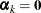). The probability of observing category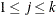is then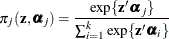For k=2, the generalized logit model reduces to a model with the logit link (a logistic model); hence the attribute generalized logit.

By default, an intercept is included in the model for the mixing probabilities. If you suppress the intercept by using the NOINT option, you must specify at least one effect in the statement.

You can specify the following probmodel-options in the PROBMODEL statement after the slash (/):

ALPHA=number

requests that confidence intervals that have the confidence level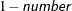be constructed for the parameters in the probability model. The value of number must be between 0 and 1; the default is 0.05. If the probability model is simple—that is, it does not contain any effects—the confidence intervals are produced for the estimated parameters (on the logit scale) and for the mixing probabilities. This option has no effect when you perform Bayesian estimation. You can modify credible interval settings by specifying the STATISTICS(ALPHA=) option in the BAYES statement.

CL

requests that confidence limits be constructed for each of the parameter estimates. The confidence level is 0.95 by default; this can be changed with the ALPHA= option.

specifies the link function in the model for the mixing probabilities. The default is a logit link for models with two components. For models with more than two components, only the generalized logit link is available. The keywords and expressions for the associated link functions for two-component models are shown in Table 39.7.

Table 39.7: Link Functions in the PROBMODEL Statement

Function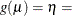CLOGLOG | CLL

Complementary log-log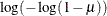LOGIT

Logit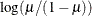LOGLOG

Log-log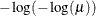PROBIT | NORMIT

Probit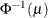NOINT

requests that no intercept be included in the model for the mixing probabilities. An intercept is included by default. If you suppress the intercept by using the NOINT option, you must specify at least one other effect for the mixing probabilities—because an empty probability model is not meaningful.

PARAMETERS(parameter-specification)
PARMS(parameter-specification)

specifies starting values for the parameters. The specification of the parameters takes the following form: parameters in the mean function appear in a list, and parameters for different components are separated by commas. Starting values are given on the linked scale, not in terms of probabilities. Also, you need to specify starting values for each of the first k1 components in a k-component model. The linear predictor for the last component is always assumed to be zero.

The following statements specify a three-component mixture of multiple regression models. The PROBMODEL statement does not list any effects; a standard intercept-only generalized logit model is used to model the mixing probabilities.

proc fmm;
model y = x1 x2 / k=3;
probmodel  / parms(2, 1);
run;


There are three linear predictors in the model for the mixing probabilities,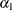,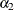, and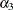. With starting values of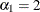,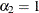, and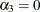, this leads to initial mixing probabilities of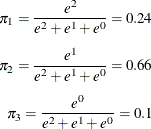You can specify missing values for parameters whose starting values are to be determined by the default method.